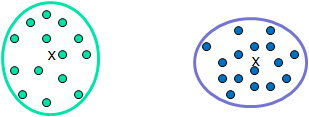# Current Slide

Small screen detected. You are viewing the mobile version of SlideWiki. If you wish to edit slides you will need to use a larger device.

### Distance between Clusters

 Single link:  smallest distance between an element in one cluster and an element in the other, i.e.,  $dist(K_{i}, K_{j}) = min(t_{ip}, t_{jq})$• Complete link: largest distance between an element in one cluster and an element in the other, i.e.,

$dist(K_{i}, K_{j}) = max(t_{ip}, t_{jq})$

• Average: avg distance between an element in one cluster and an element in the other, i.e.,

$dist(K_{i}, K_{j}) = avg(t_{ip}, t_{jq})$

• Centroid: distance between the centroids of two clusters, i.e.,

$dist(K_{i}, K_{j}) = dist(C_{i}, C_{j})$

• Medoid: distance between the medoids of two clusters, i.e.,
$dist(K_{i}, K_{j}) = dist(M_{i}, M_{j})$
• Medoid: a chosen, centrally located object in the cluster

Speaker notes:

## Content Tools

### Sources

There are currently no sources for this slide.